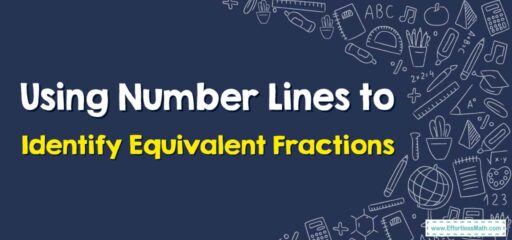# How to Use Number Lines to Identify Equivalent Fractions?

Fractions that have different numerators and denominators but are equal to the same value are equivalent fractions. In this step-by-step guide, you can learn how to use number lines to identify equivalent fractions.## A Step-by-step guide to using number lines to identify equivalent fractions

Two or more fractions that show the same parts of a whole are called equivalent fractions. Equivalent fractions always represent the same point on the number line. There are two ways to indicate equivalent fractions: 1-Fraction bars, and 2-Number line.

In the Number line method, we draw a number line with whole numbers to represent any number. Then, we divide the space between each whole into equal parts, and the number of equal parts must match the number in the denominator. Then specify the point equal to the number.

### Using Number Lines to Identify Equivalent Fractions-Example 1:

What is the equivalent fraction of $$\frac{1}{2}$$?

Solution: First, draw a number line of $$0$$ and $$1$$. Then divide the total into two parts equal to the denominator. Then check line $$\frac{1}{2}$$. Then divide each part into two equal parts. Then for the same situation, we can see that the new fraction is $$\frac{2}{4}$$ because there are $$4$$ equal parts and the marking is after two equal parts. So, $$\frac{1}{2}$$ and $$\frac{2}{4}$$ are equivalent fractions.

## Exercises forUsing Number Lines to Identify Equivalent Fractions

### Use a number line to find an equivalent fraction.

1. $$\color{blue}{\frac{1}{4}}$$
2. $$\color{blue}{\frac{2}{4}}$$

1.

2.

### What people say about "How to Use Number Lines to Identify Equivalent Fractions? - Effortless Math: We Help Students Learn to LOVE Mathematics"?

No one replied yet.

X
30% OFF

Limited time only!

Save Over 30%

SAVE $5 It was$16.99 now it is \$11.99